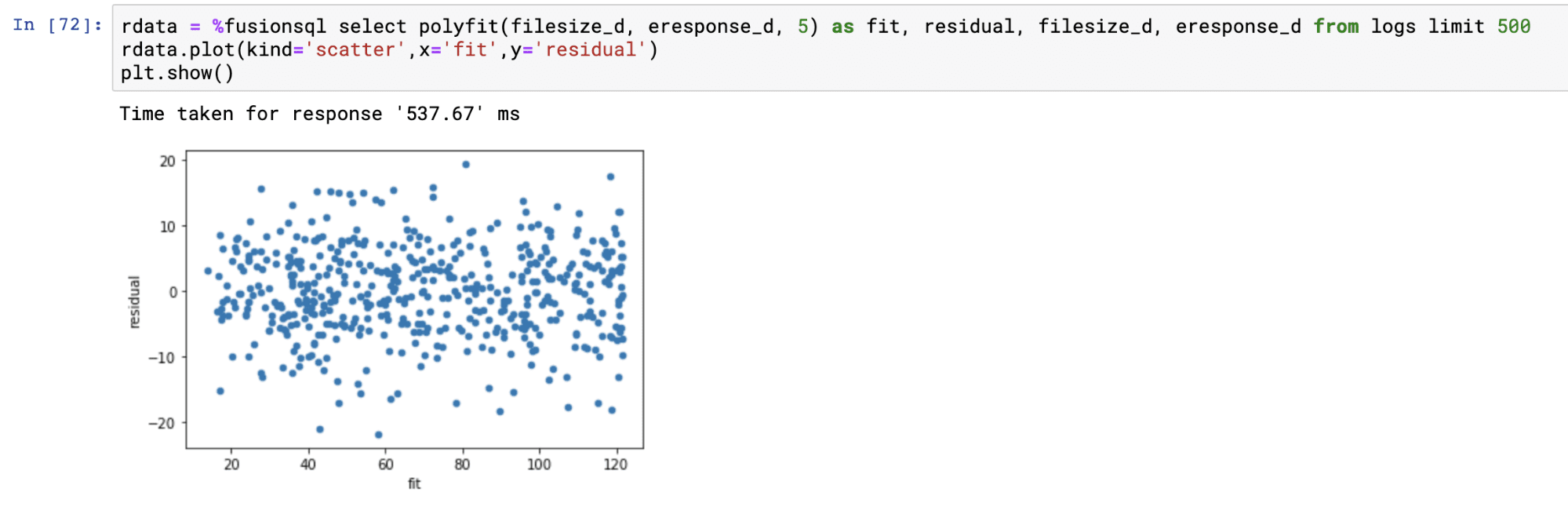# Use Jupyter with Fusion SQL

This guide provides basic setup steps for connecting to Fusion SQL in a Jupyter notebook, then shows examples of how to query and visualize result sets and statistical functions from Fusion SQL. The full scope of Fusion SQL query capabilities is covered in Querying Fusion SQL.

## Basic setup

1. Navigate to https://<fusion-domain>/jupyter in your browser.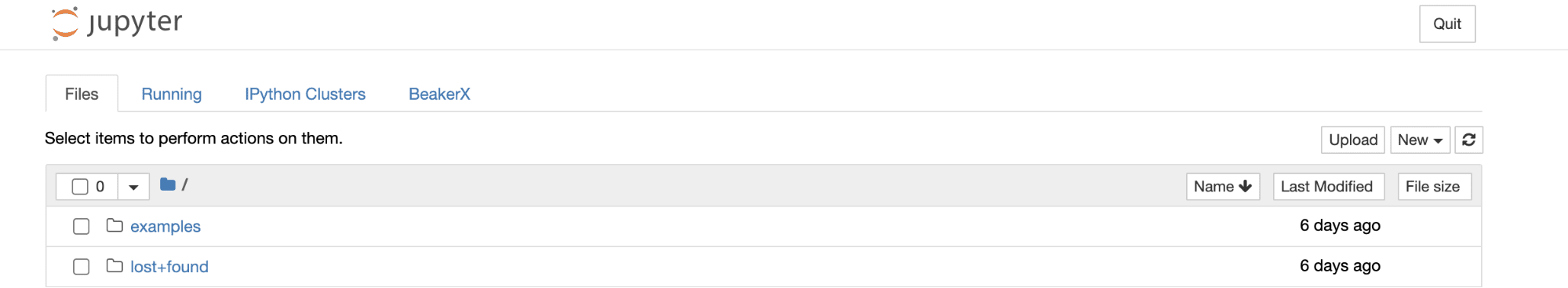2. Create a new Python 3 notebook: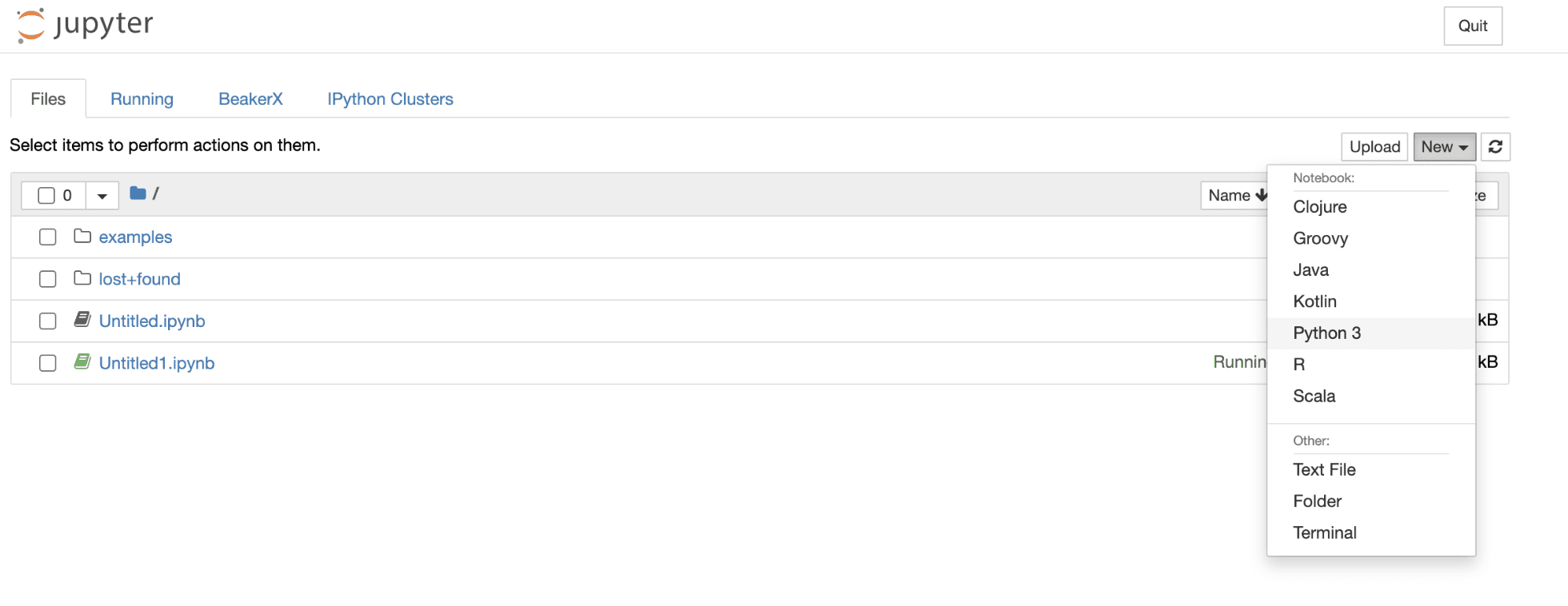3. Connect to Fusion SQL through the Fusion proxy by issuing the following commands into the notebook: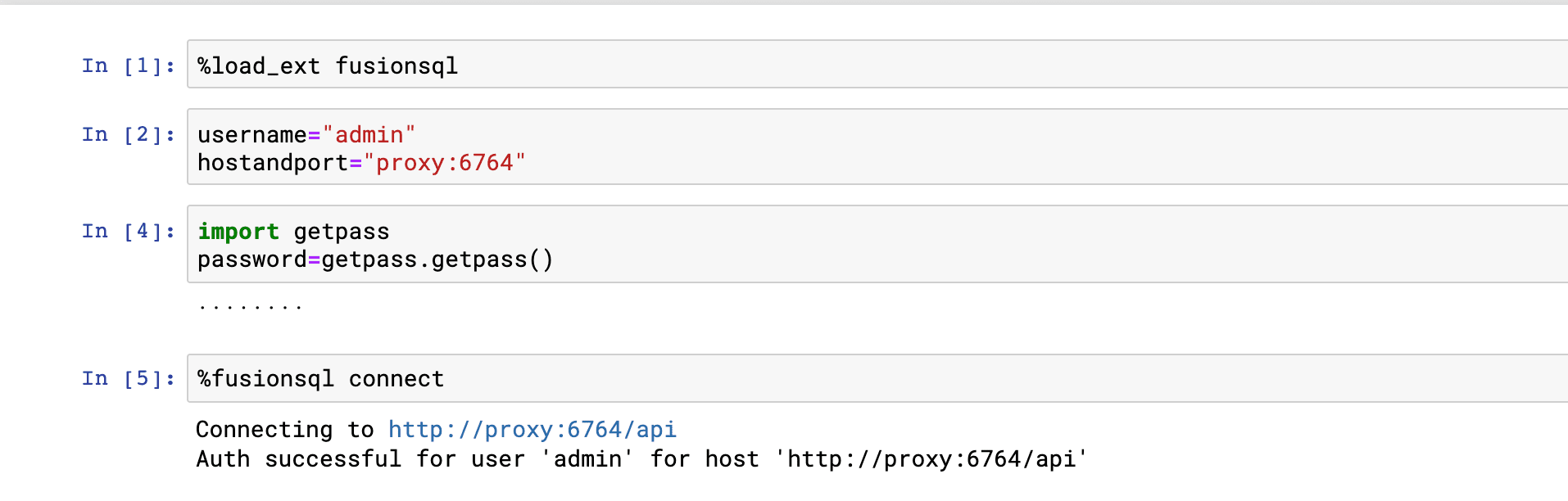## Showing and describing tables

The `fusionsql` Python extension is used to issue commands and SQL queries to Fusion SQL.

The `show tables` command can be used to list the available tables: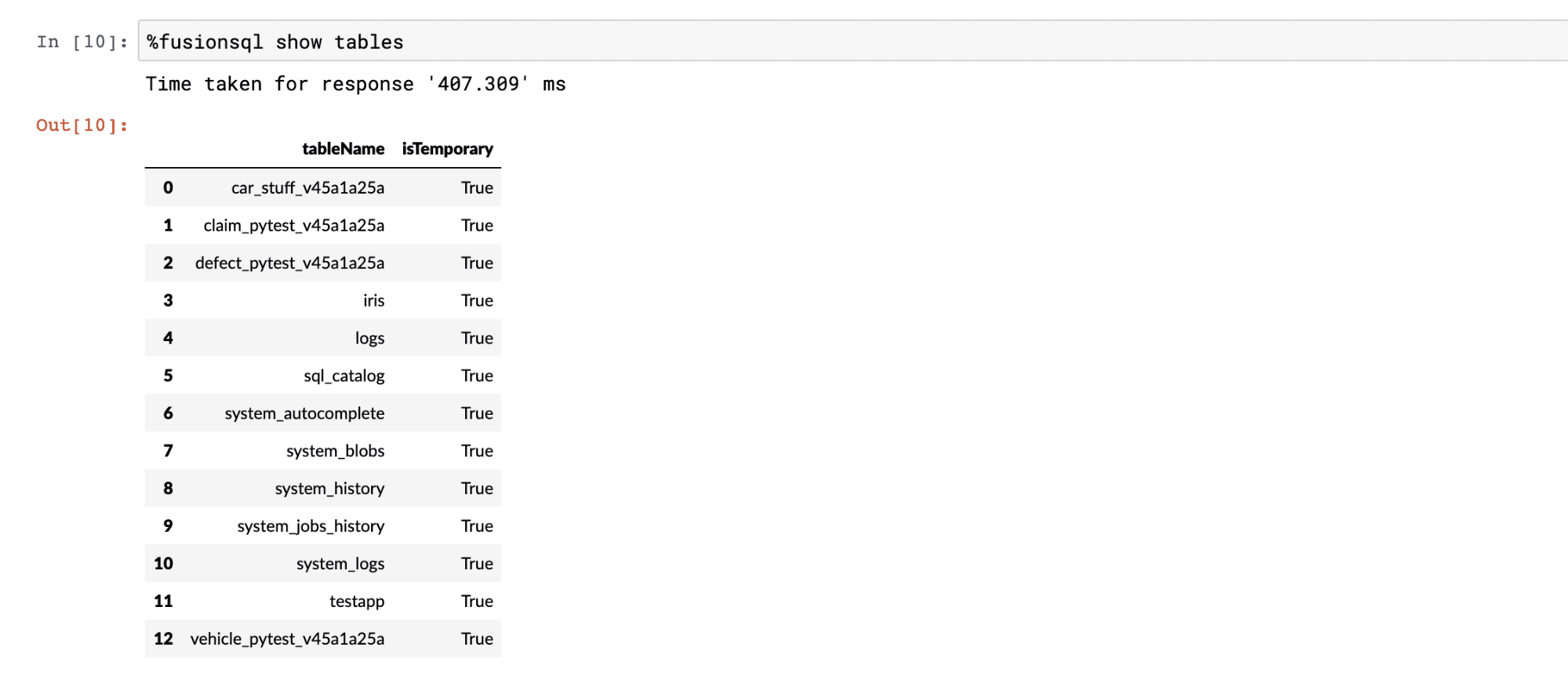The `describe tablename` command can be used list the columns and data types in a table: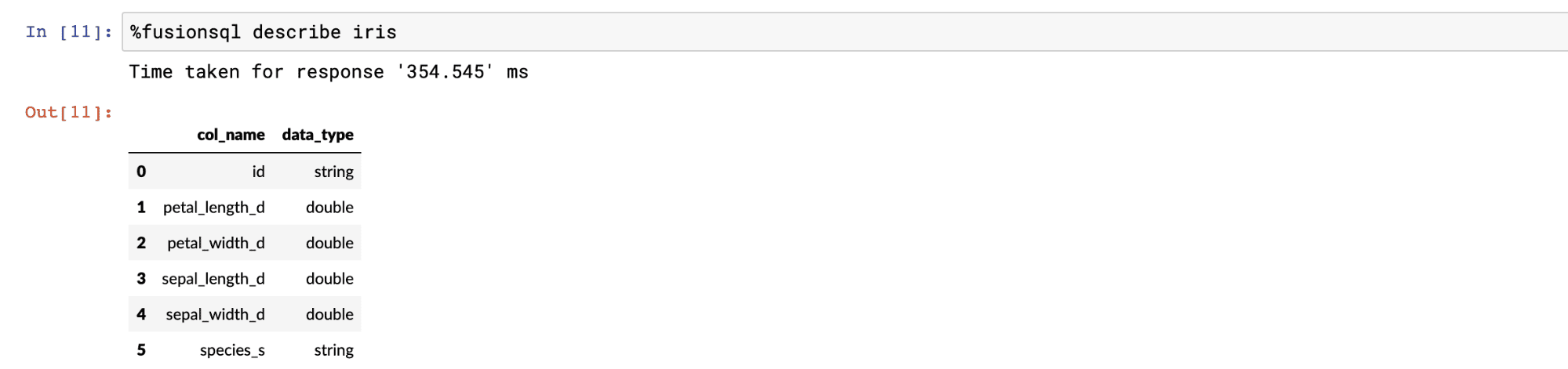## Querying with SQL

SQL is used to query the data in the tables. All SQL queries return a Pandas dataframe. If the result set is not set to a variable it is displayed in the notebook. The example below shows a SQL query and output: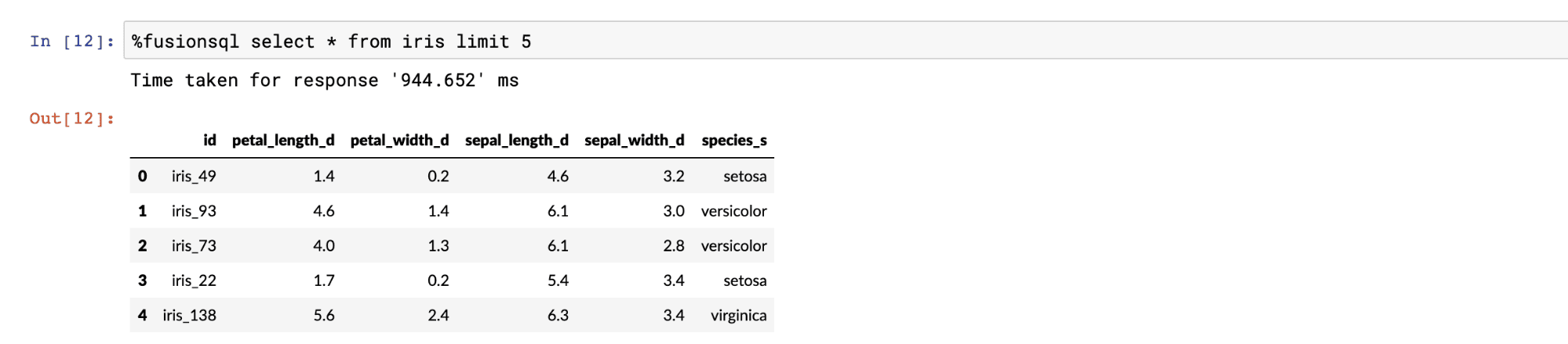## Sampling and visualization

Basic select queries that do not include an order by return a random sample that matches the result set. Fusion SQL has very fast and scalable sampling capabilities. Sampled result sets can be set to variables which are Pandas dataframes. The result sets can be manipulated and visualized through standard dataframe functions.

The example below issues a SQL query and sets it to the variable data, which is a Pandas dataframe. The next statement calls the `boxplot` function directly on the dataframe. The boxplot appears below. Boxplots allow for the quick visual comparison of the distributions within the columns in the dataframe.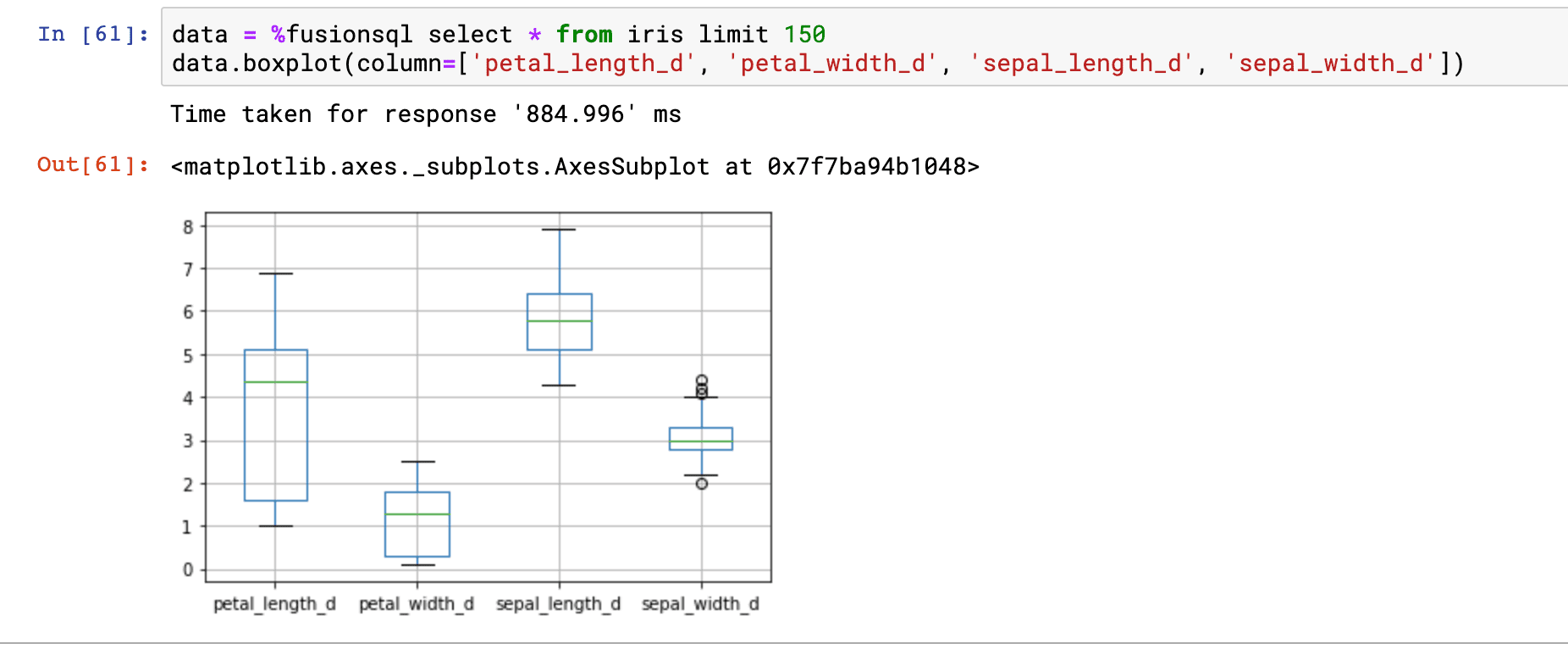In the example above we’re comparing the distributions of the `petal_length_d`, `petal_width_d`, `sepal_length_d` and `sepal_width_d` fields.

This result set also contains a categorical field called `species_s`. We can change the orientation of the dataframe so that we can do a boxplot of the `petal_length_d` field by the `species_s` field. The dataframe has a `pivot` function which can change the orientation of the result set so that the values in the `species_s` field become the column headers of the dataframe. Below is an example of how to use the `pivot` function: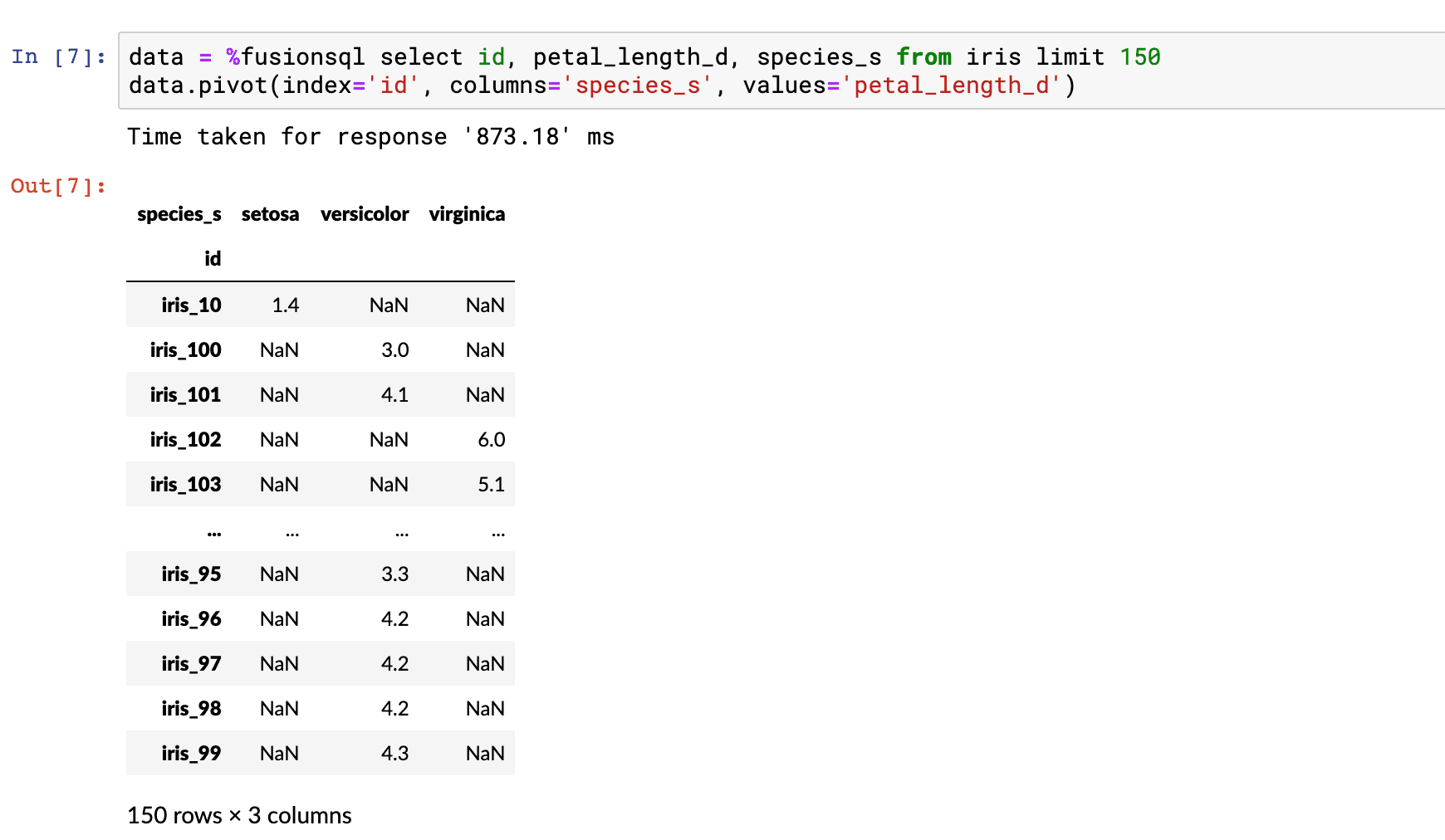Notice that we now have a matrix with the `species_s` field as column headers. Each row in the table only has `petal_length_d` data populated for one of the `species_s` types.

The `boxplot` function conveniently ignores the NaN values to create a boxplot for each `species_s` column.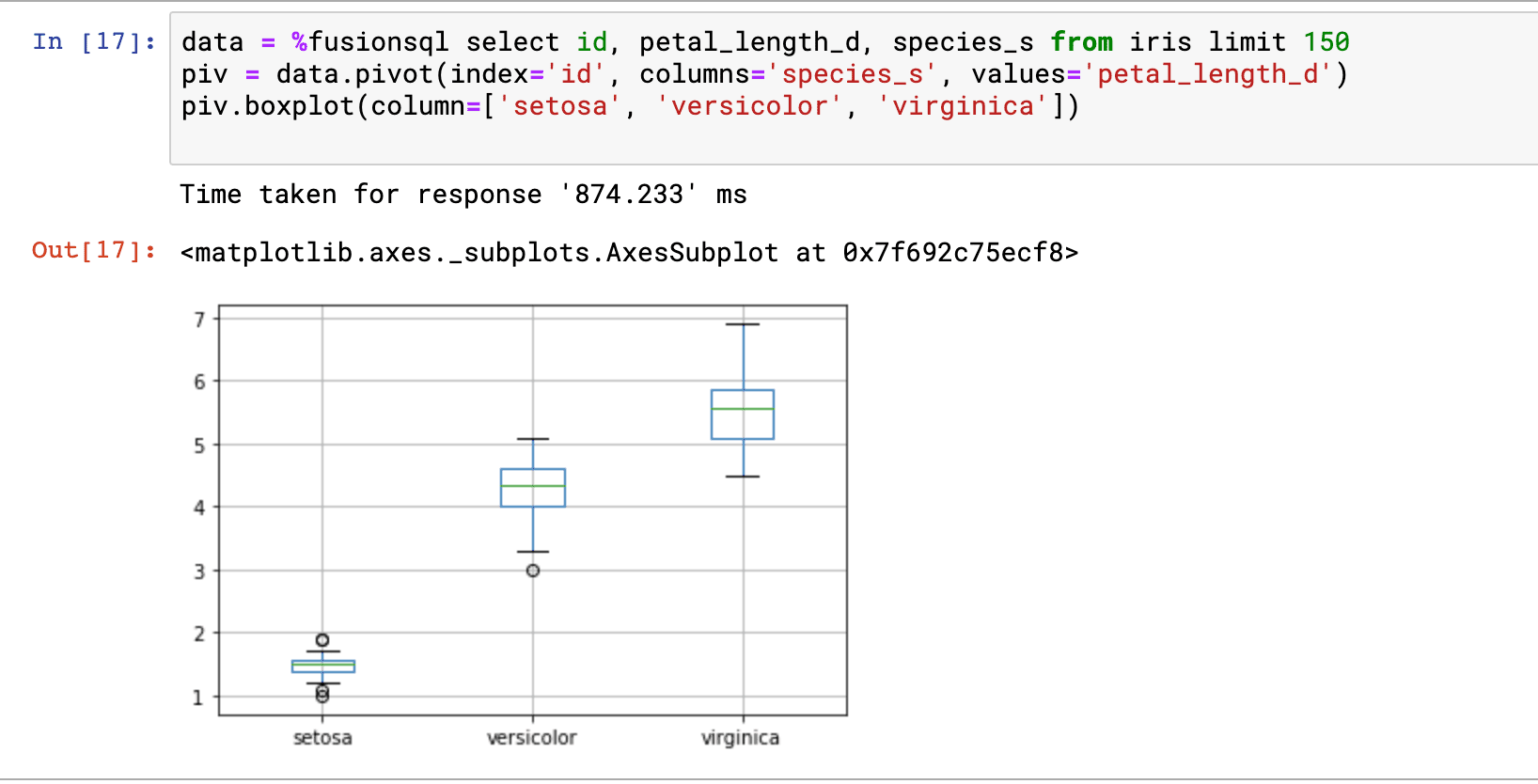Samples can also be used to visualize the relationship between two numeric fields. The example below takes a sample and sets it to a dataframe. The dataframe `plot` function is then used to plot a scatter plot with `petal_length_d` on the x-axis and `petal_width_d` on the y-axis. From this plot we can quickly see how `petal_length` affects `petal_width`.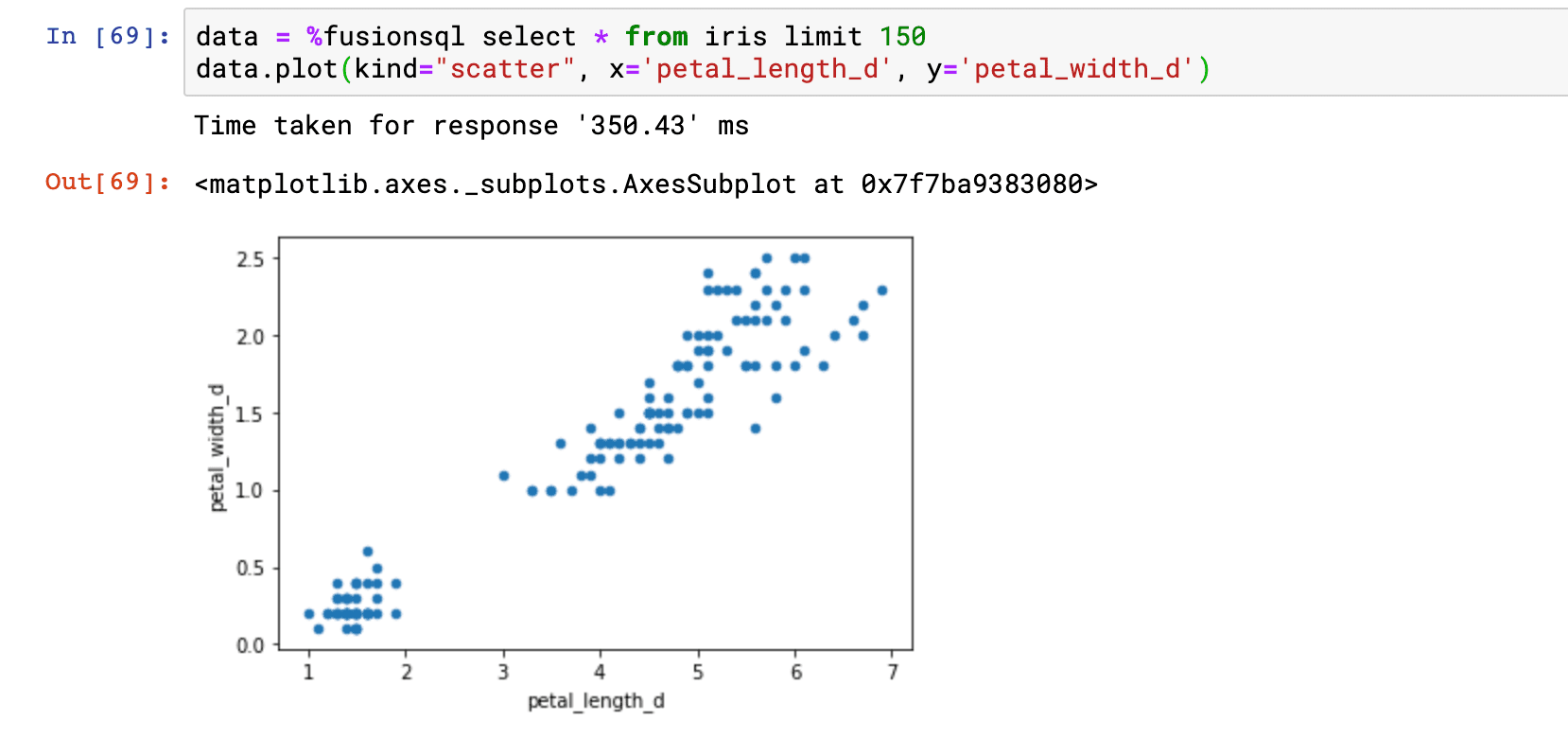We can also add the `species_s` label to the visualization and color the points by species. This allows us to see the relationship between the two variables by species.

In order to add colored labels we’ll use the Seaborn Python package. In the example below the Seaborn package is imported and then the `lmplot` function is used to plot the labeled and colored scatter plot.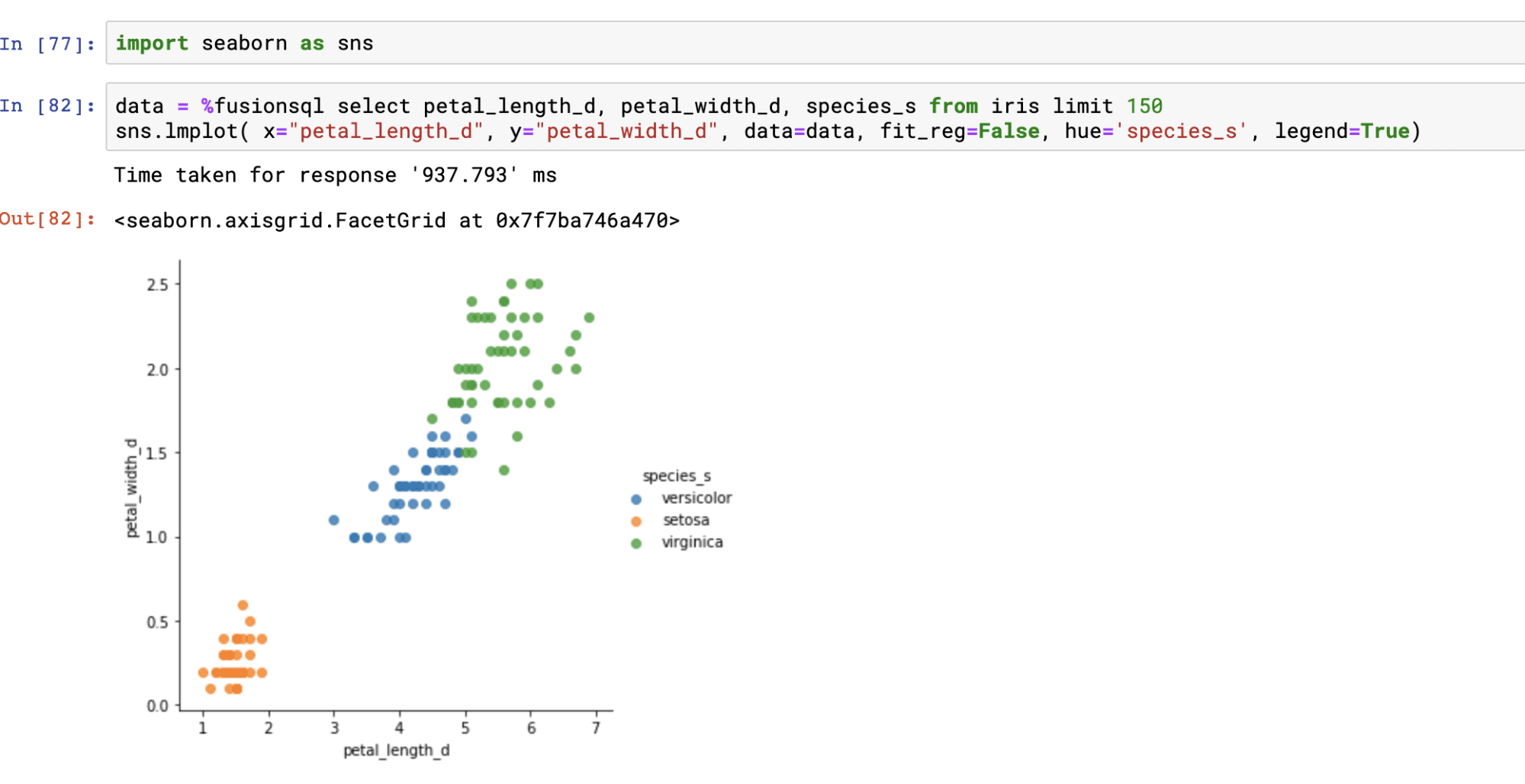## Descriptive statistics

Descriptive statistics for a result set can be calculated using standard SQL.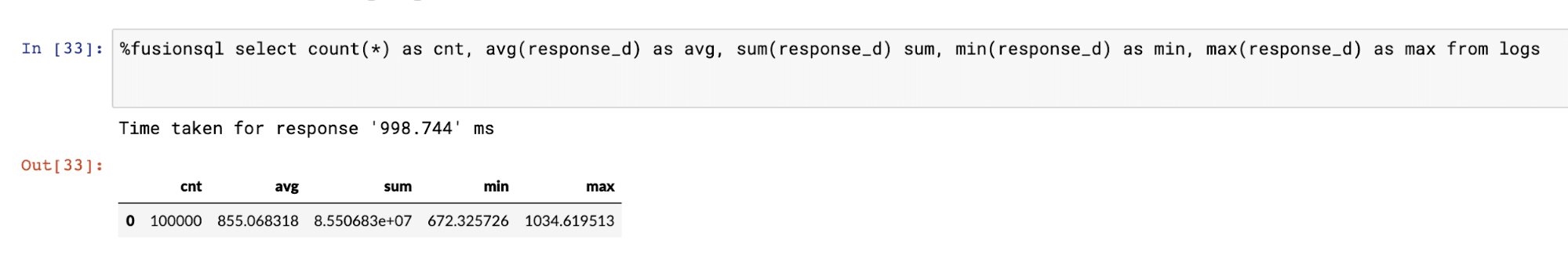## Aggregations and visualizations

Single and multi-dimensional aggregations can be calculated using `GROUP BY SQL` queries.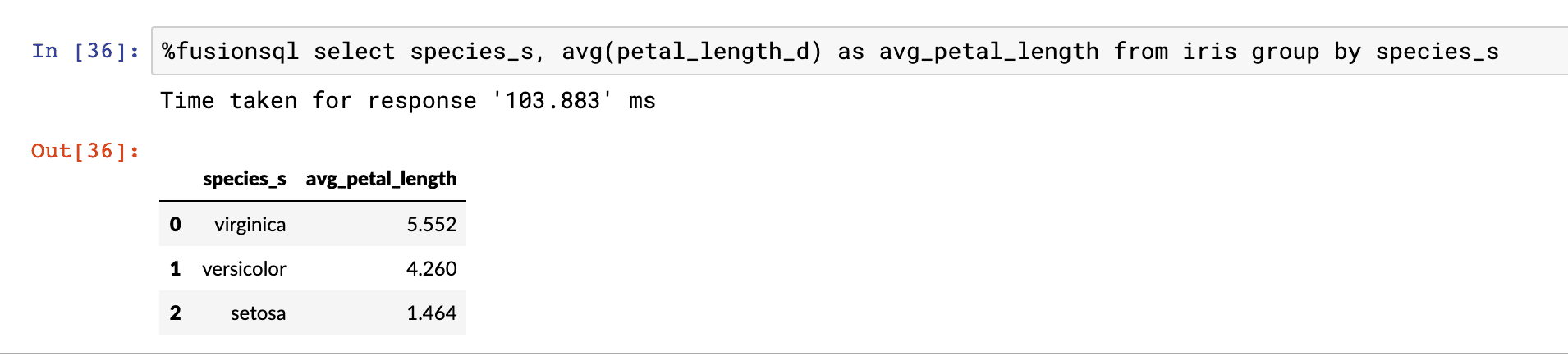The result of aggregations can be set to a Pandas dataframe and visualized: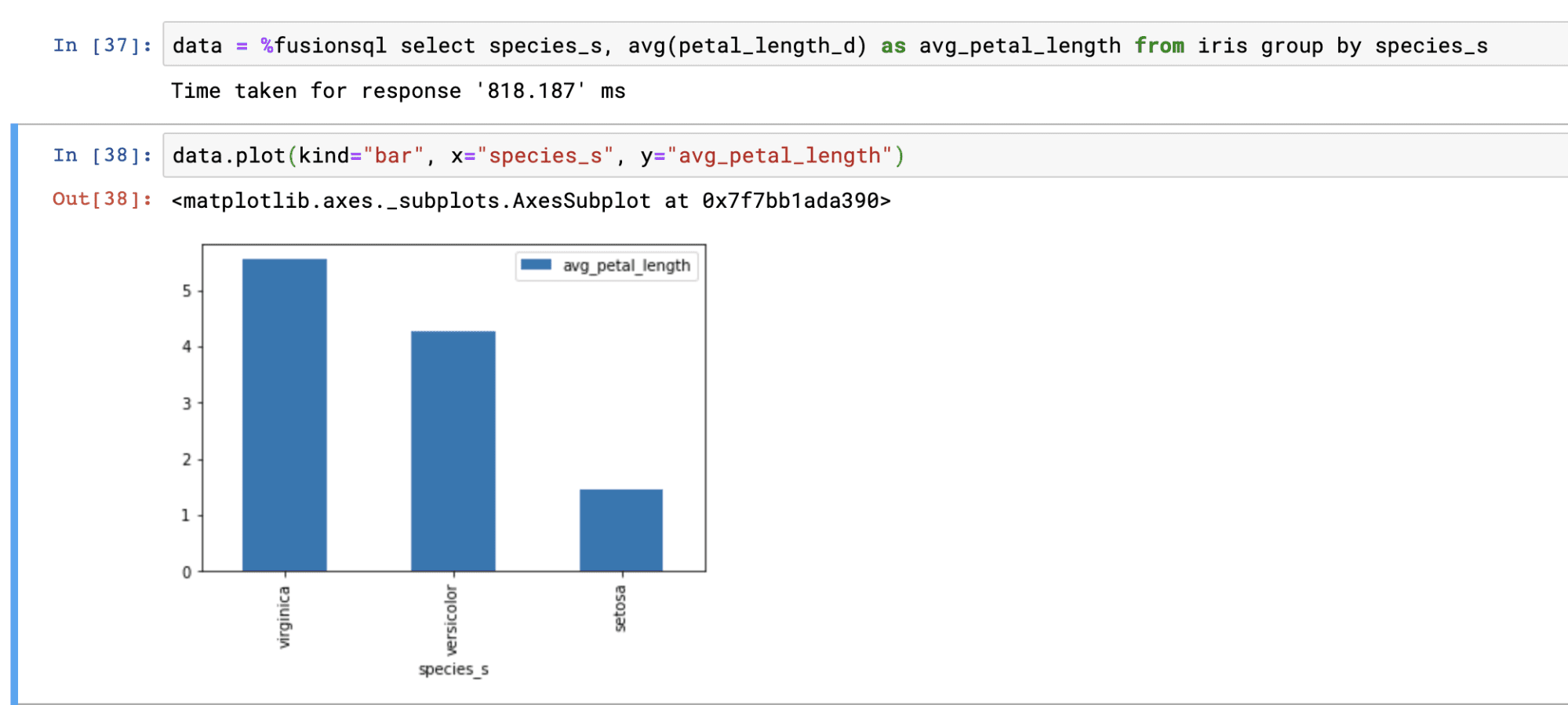## Time series and visualization

Fusion SQL has a powerful time series extension implemented through the SQL `date_format` function. The `date_format` function allows for efficient grouping over datetime fields. The `date_format` function also allows for the specification of the output format and the gap.

The example below shows the output of a `GROUP BY` query over the `date_format` function.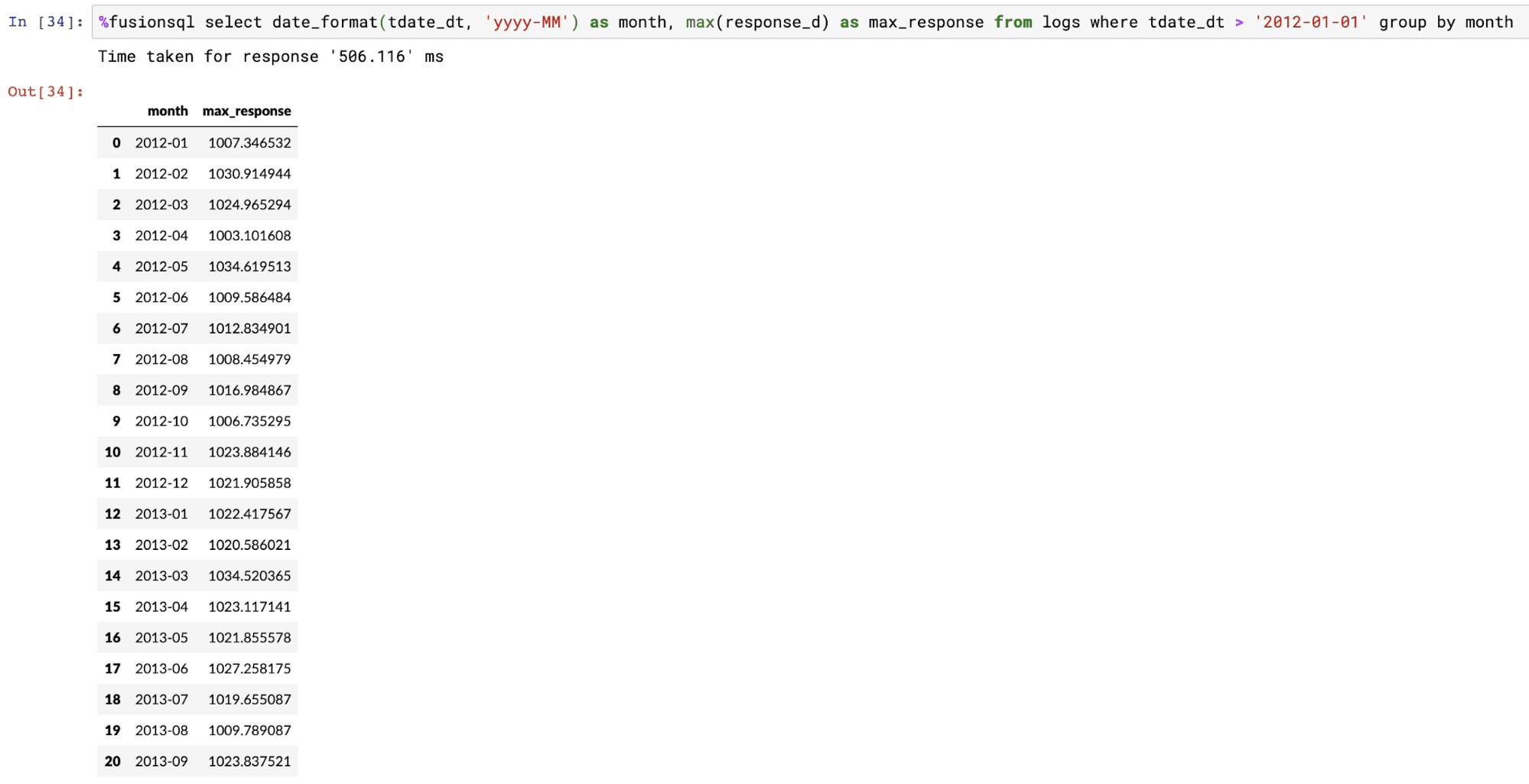The example below shows the time series result set plotted on a line chart using the dataframe `plot` function.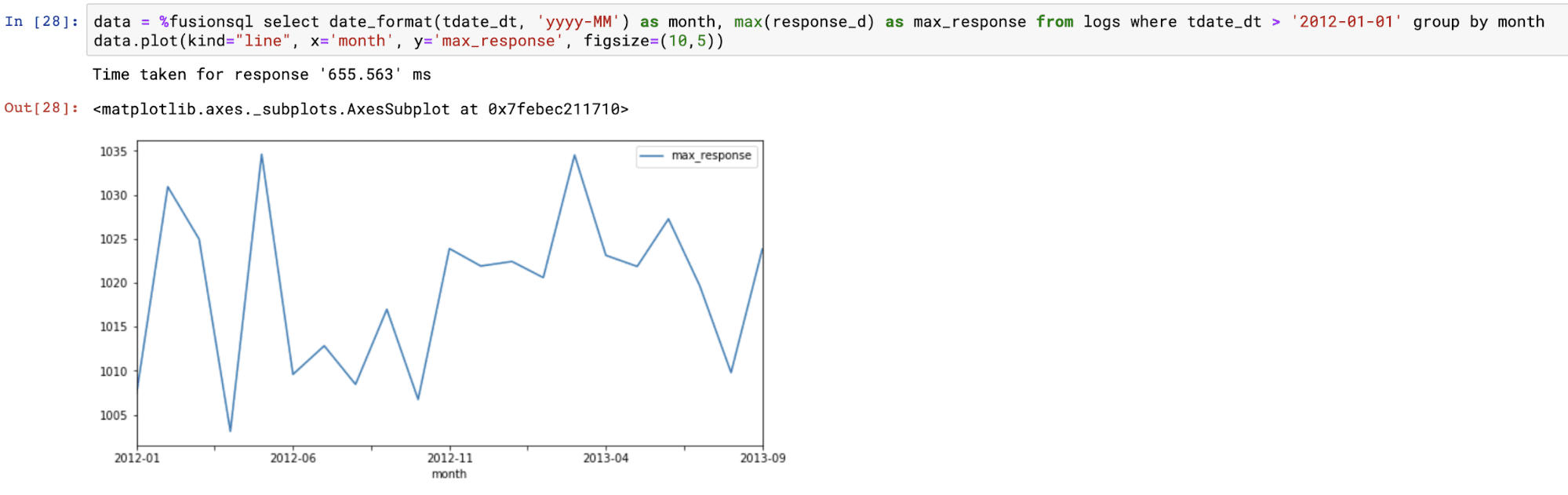## Statistical analysis and visualizations

Fusion SQL includes a library of functions designed for statistical analysis and visualization. The section below demonstrates a subset of these functions within Jupyter notebook.

### Histograms

Histograms can be created from a numeric field using the `hist` function. The `hist` function takes three parameters:

• The numeric field to create the histogram from

• The number of bins in the histogram

• The sample size

The hist function returns the mean of each bin. There are three other fields available for selection when the `hist` function is used:

• `hist_count`: The number of samples within each bin.

• `hist_prob`: The probability that sample will fall within each bin.

• `hist_cum_prob`: The cumulative probability for each bin.

Below is an example of the `hist` function visualized in an area chart with the `hist_mean` on the x-axis and the `hist_count` on the y-axis: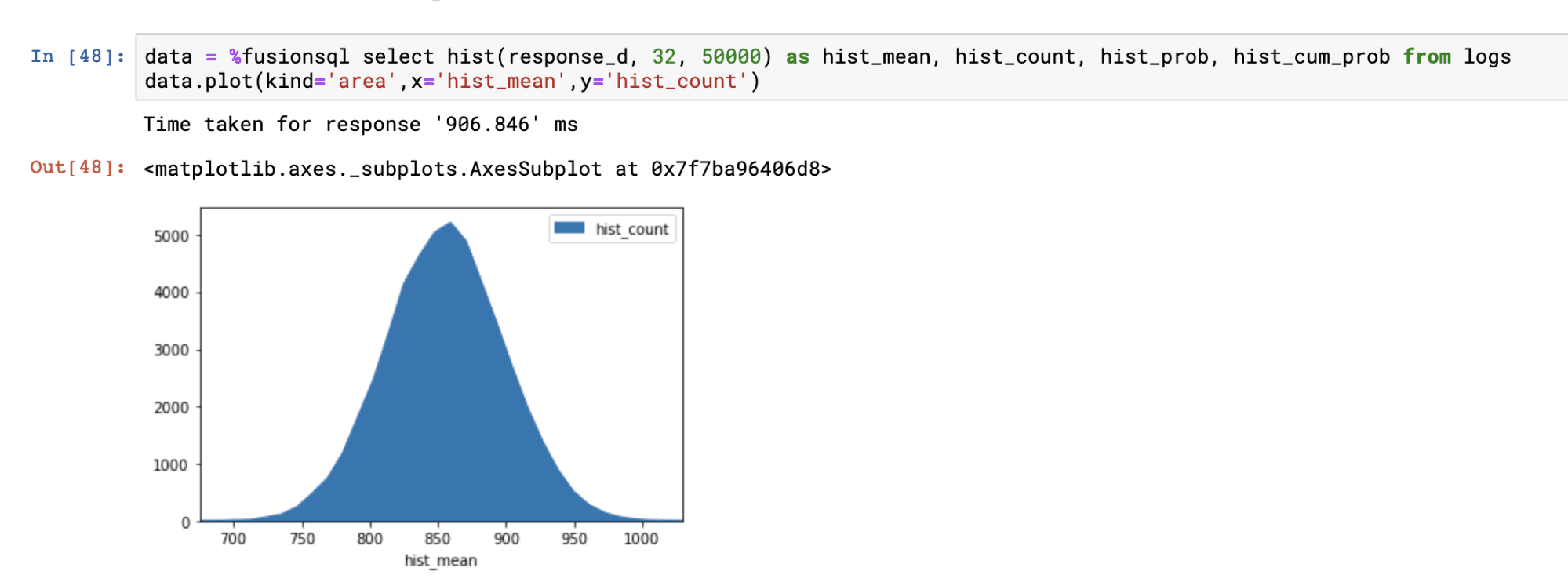Below is an example with `hist_cum_prob` plotted on the y-axis: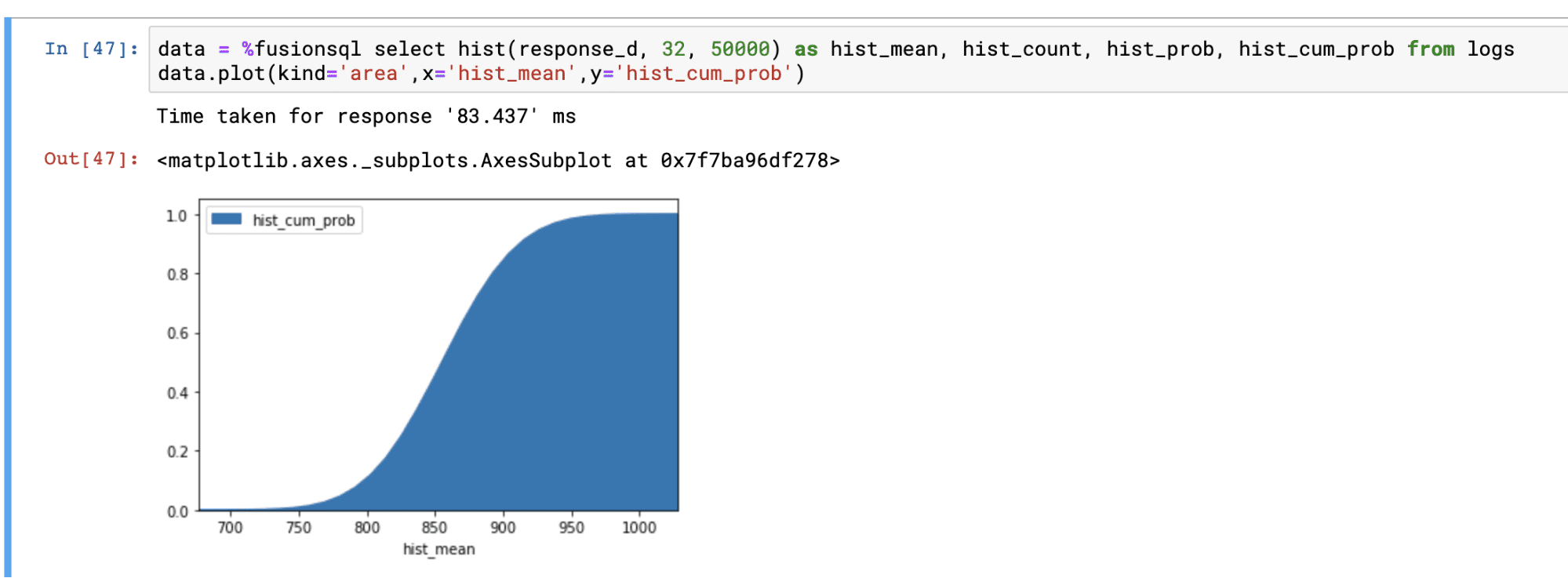### Fitting a gaussian curve to a histogram

The `gaussfit` function fits a gaussian curve to a histogram. You would do this to visualize how well the data in a numeric field fits a normal distribution.

The `gaussfit` function takes two parameters:

• The numeric field to create the histogram from

• The sample size

The `gaussfit` function returns the fitted curve at each bin in the histogram. There are two additional fields that can be selected when the `gaussfit` function is used:

• `hist_bin`: The bin number for the histogram

• `hist_count`: The count of the number of samples for each bin

The example below shows the histogram and fitted curve plotted on the same figure. The example demonstrates how to run the `gaussfit` function and how to overlay the fitted curve on top of the histogram.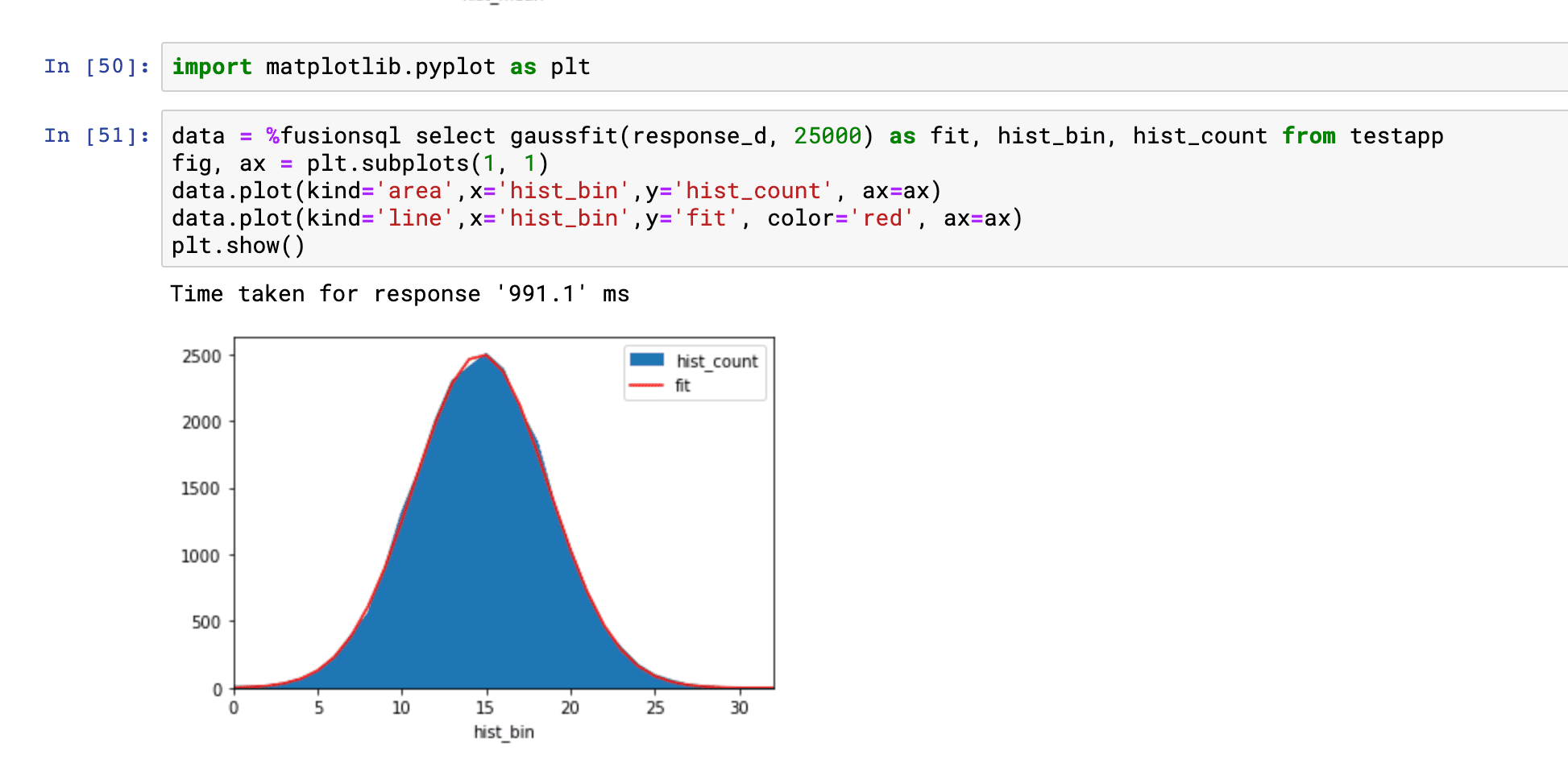### Percentiles

The `percentiles` function calculates percentile estimates for a numeric field. The `percentiles` function takes two parameters:

• The numeric field to calculate the percentiles from

• The sample size

The `percentiles` function returns the percentile (1-99). The `percentiles_estimate` field returns the estimated percentile value for each percentile.

Below is an example of the `percentiles` function plotted on a line chart: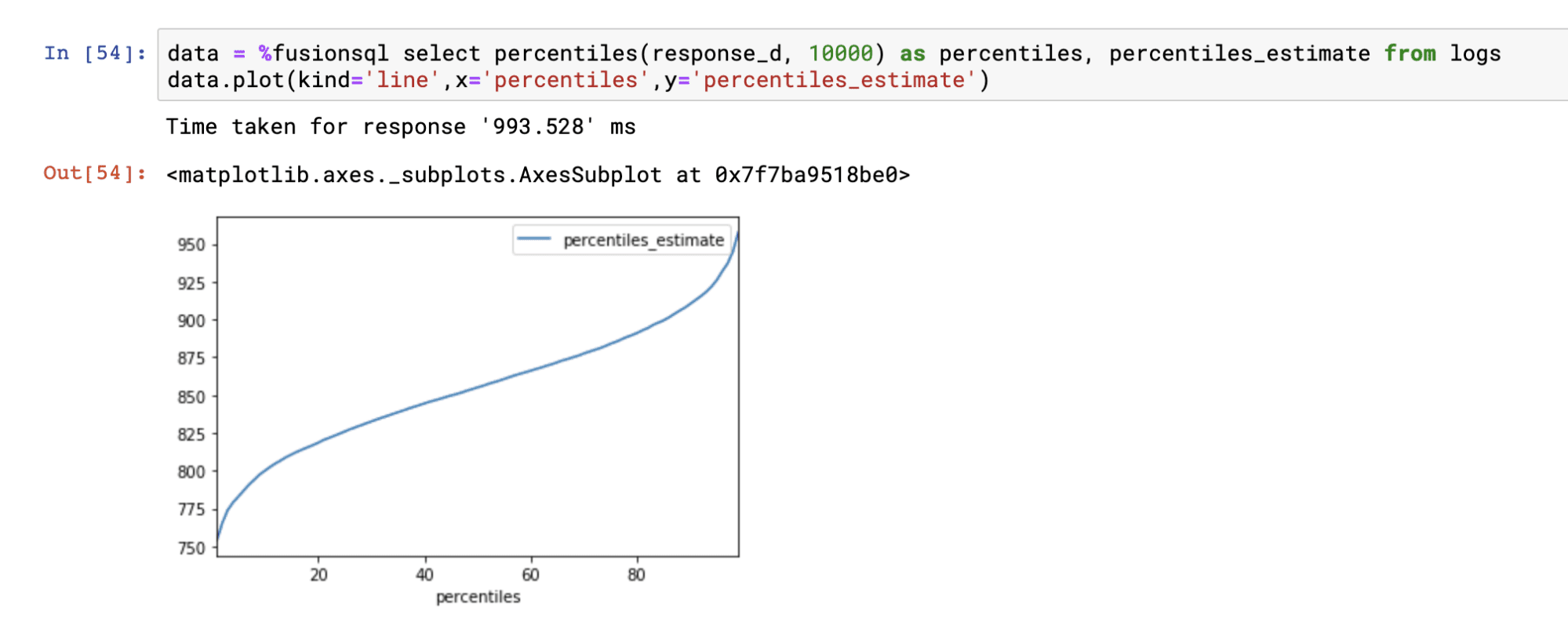### Correlation matrices

Correlation matrices can be computed using the `corr_matrix` function. The `corr_matrix` function takes two parameters:

• A single quoted string containing a comma delimited list of fields to build the correlation matrix from

• The sample size

The `corr_matrix` function returns the Pearson’s correlation coefficient for each combination of the fields. The `matrix_x` and `matrix_y` fields contain the x and y labels of the matrix.

The example below shows the output of the `corr_matrix` function: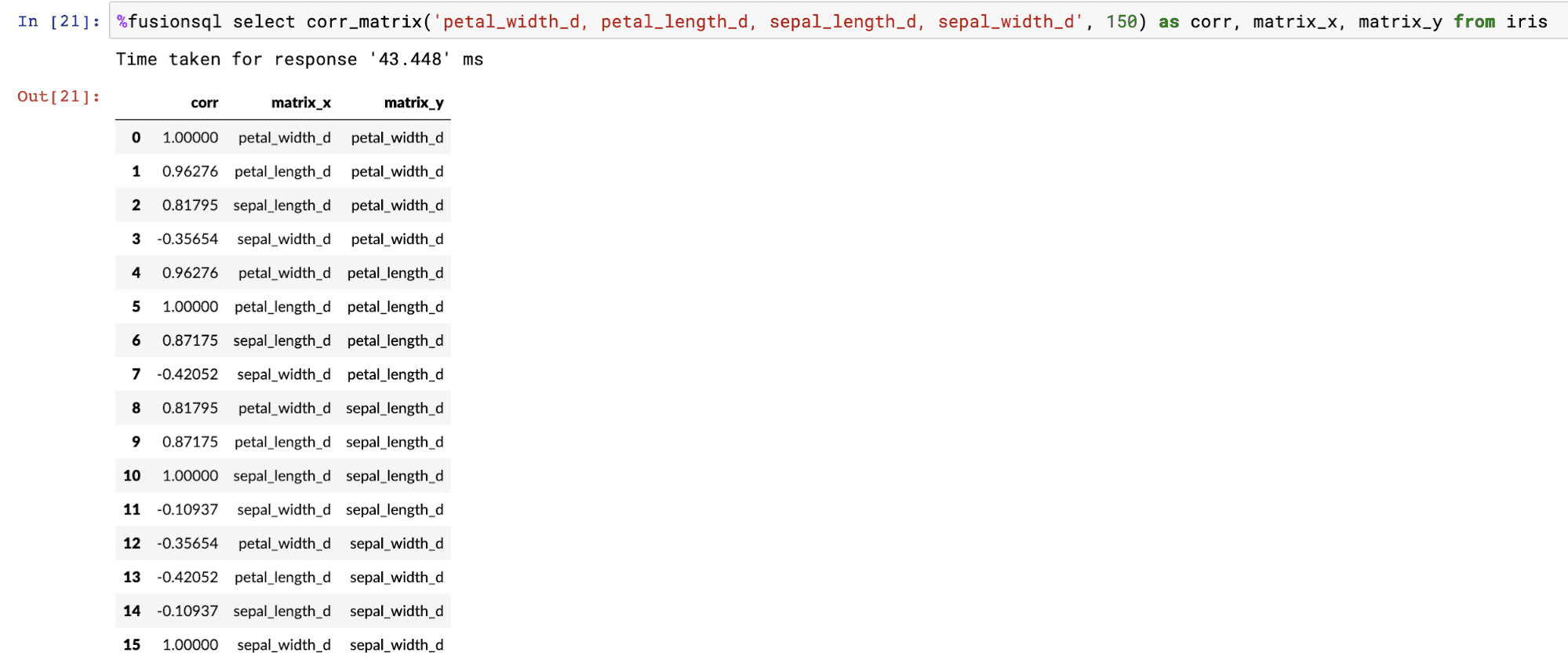The dataframe created by the `corr_matrix` function can be pivoted into a matrix using the `pivot` function: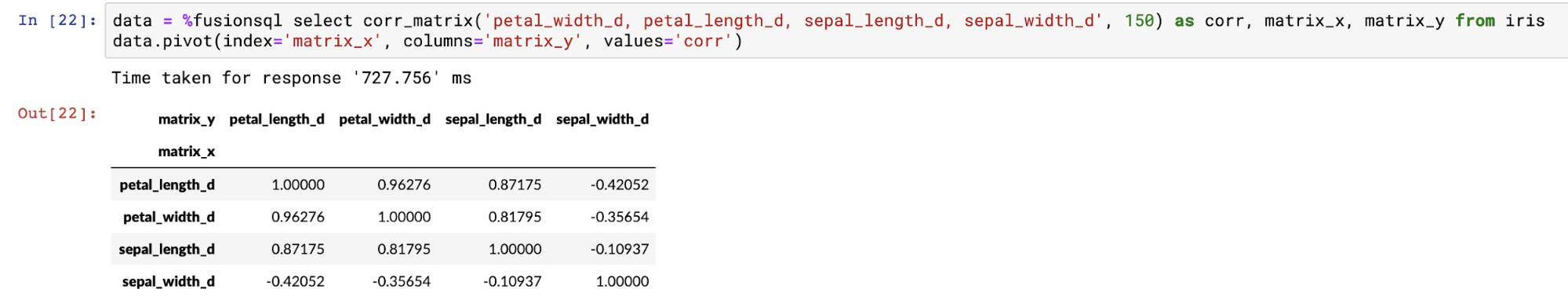Once pivoted, the Matplotlib `pcolor` function can be used to display the correlation matrix as a heat map. Below is an example of how to visualize the the `corr_matrix` function: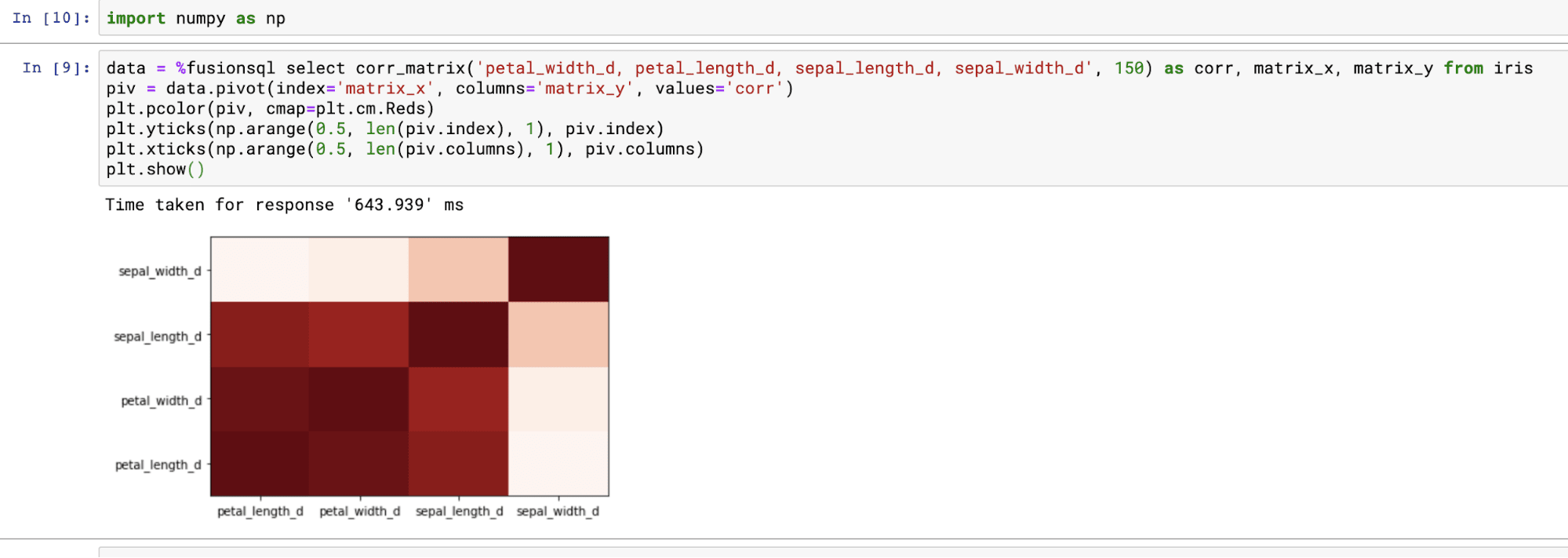### Linear regression and residual plots

The `linearfit` function can be used to visualize bi-variate linear regression plots and residual plots. The `linearfit` function takes two parameters:

• The numeric predictor or independent variable field (x).

• The numeric dependent variable field (y).

The `linearfit` function returns the predicted value for each of the predictor values. There are also three other fields which can be selected in the result set:

• The numeric predictor or independent variable field

• The numeric dependent variable field

• residual : The difference between the actual value and the predicted value. This represents the error for each prediction.

Using predicted values and the residual values the regression and residual plots can be visualized.

The example below shows the regression plot. The regression plot is drawn by first plotting the dependent and independent variables in scatter plot. Then the predicted values are overlaid through the scatter to visualize how well the line fits through the points.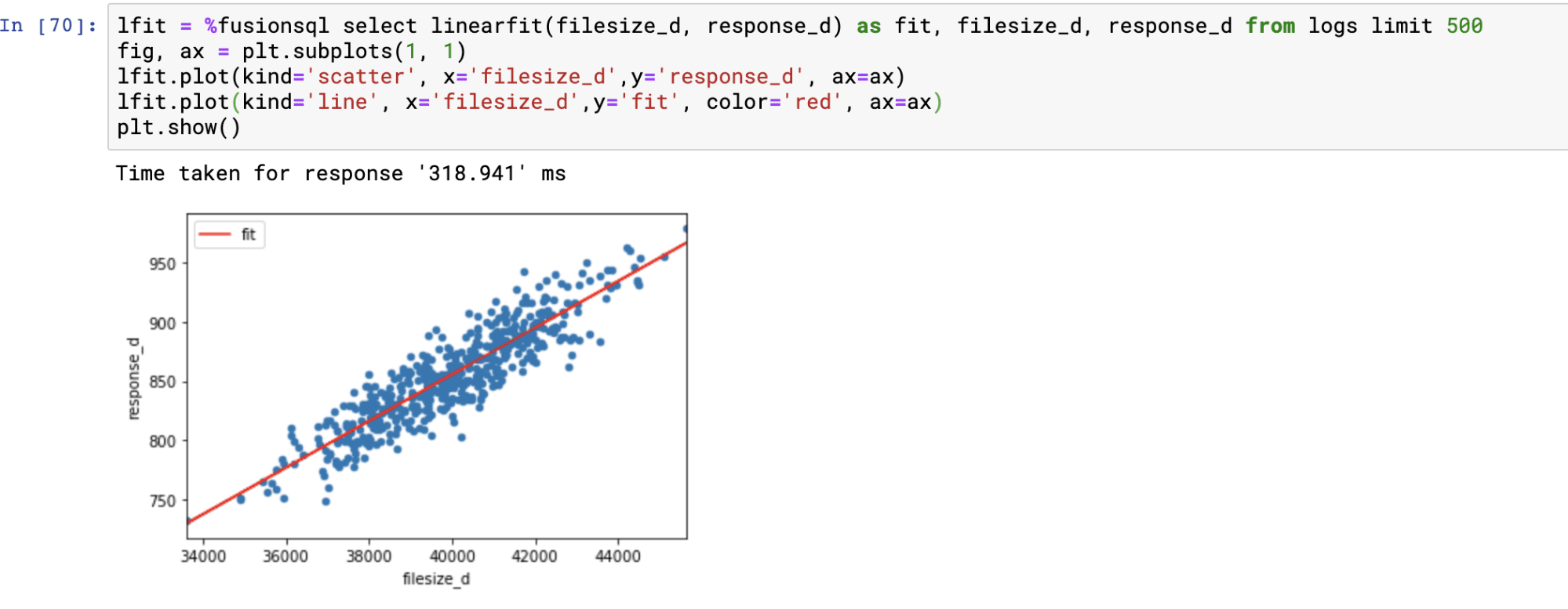The residual plot is done by plotting the predictions on the x-axis and the residual values on the y-axis. The residual plot visualizes the error across the full range of predictions.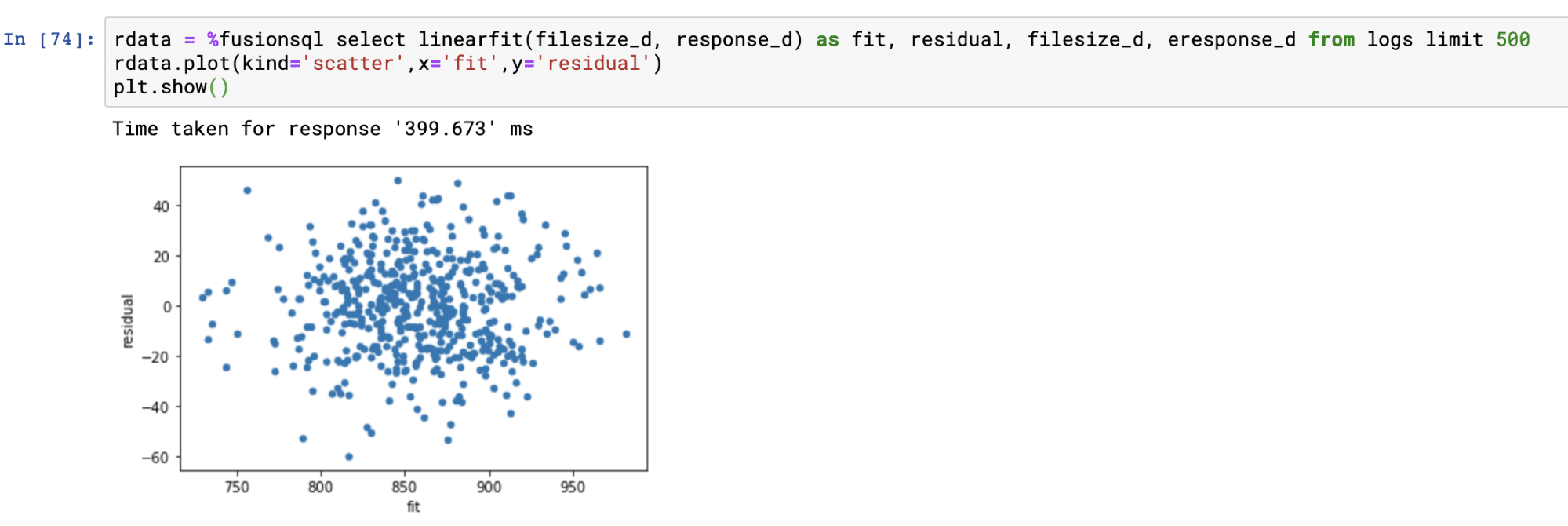### Polynomial non-linear regression

The `polyfit` function fits a smooth curve through a bi-variate scatter plot. The `polyfit` function works similarly to the `linearfit` function except it has one extra parameter which specifies the degree of the polynomial used to fit the curve. The higher the degree, the larger the number of curves. The degree is typically an integer between 1 and 5.

Below is an example of a regression plot using the `polyfit` function with a 5 degree polynomial. The only difference in the plotting technique from `linearfit` is that the predictions are plotted with a scatter plot. See `linearfit` for more details on regression plots.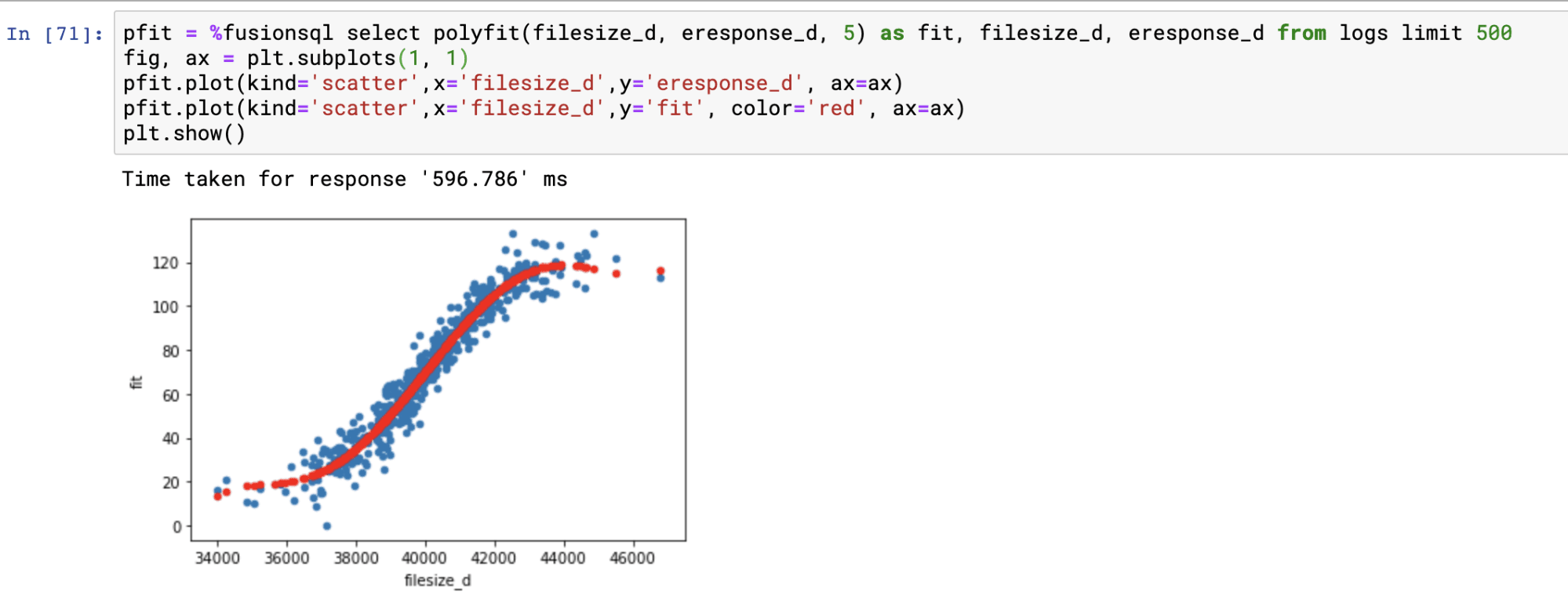The residual plot is handled in exactly the same manner as with the `linearfit` residual plot.